Select Page

# Digital Modulation Techniques

Digital Modulation Techniques

Contents

Recall the Digital Transmission of Data 3

List various Digital Modulation Techniques 3

Describe the block diagram of ASK Transmitter 4

Recall the Bandwidth and Energy in ASK Transmission 6

Draw Constellation diagram of ASK 6

Describe the block diagram of FSK Transmitter 9

Recall the Bandwidth and Energy in FSK Transmission 11

Draw Constellation diagram of FSK 12

Describe the block diagram of FSK Receiver 13

Describe the block diagram of PSK Transmitter 15

Recall the Bandwidth and Energy in PSK Transmission 16

Draw Constellation diagram of PSK 16

Describe the block diagram of PSK Receiver 17

Describe the block diagram of QPSK Transmitter 19

Recall the Bandwidth and Energy in QPSK Transmission 20

Draw Constellation diagram of QPSK 20

Describe the block diagram of QPSK Receiver 21

Recall the block diagram of DPSK Transmitter 23

Recall the block diagram of DPSK Receiver 24

Compare 16-ary QASK with 16-ary PSK 26

Explain Continuous Phase Modulation 28

Describe Minimum Shift Keying (MSK) 29

Describe Gaussian MSK (GMSK) 31

Describe the Spread Spectrum Communication 32

Recall the types of Spread Spectrum Communication 33

Describe Intersymbol Interference 33

Recall Nyquist Criterion for zero ISI 34

Recall the method by which ISI can be controlled 35

Describe Code Division Multiple Access(CDMA) 35

Recall the principle of DSSS-CDMA 36

Recall the concept of Near-far problem and power control in CDMA 37

Recall the Applications of CDMA Technology 37

# Recall the Digital Transmission of Data

Digital transmission of data refers to the process of transmitting digital signals or data over a channel or link, using a suitable digital communication technique or system. Digital transmission of data is an important aspect of modern communication systems, as it enables the transmission of digital information such as text, images, audio, or video, over a wide range of distances and environments.

Digital transmission of data has several advantages over analog transmission of data, such as higher accuracy, reliability, and efficiency. Digital signals can be transmitted with a high degree of accuracy, as they can be represented by a finite number of discrete levels or states. Digital signals are also less sensitive to noise or interference, as they can be transmitted using error-correction or error-detection techniques. Digital transmission of data is also more efficient, as it allows for the transmission of more data in a given bandwidth or time, compared to analog transmission of data.

There are several types of digital transmission of data, such as pulse code modulation (PCM), time division multiplexing (TDM), frequency division multiplexing (FDM), and spread spectrum. Each type of digital transmission of data has its own characteristics and trade-offs, and is suitable for different applications and environments. For example, PCM is used to transmit digital signals with a wide range of amplitudes or frequencies, TDM is used to transmit digital signals with a high data rate or capacity, FDM is used to transmit digital signals over a wide bandwidth or frequency range, and spread spectrum is used to transmit digital signals over a noisy or crowded channel or link.

# List various Digital Modulation Techniques

There are several digital modulation techniques that are used to transmit digital signals over a channel or link, such as:

1. Pulse code modulation (PCM): PCM is a digital communication technique that is used to transmit digital signals with a wide range of amplitudes or frequencies. PCM encodes the input digital signal into a series of pulses, which are modulated onto a suitable carrier frequency using a technique called pulse code modulation (PCM).

2. Time division multiplexing (TDM): TDM is a digital communication technique that is used to transmit digital signals with a high data rate or capacity. TDM divides the available bandwidth or time into a series of time slots or channels, and assigns each time slot or channel to a different digital signal.

3. Frequency division multiplexing (FDM): FDM is a digital communication technique that is used to transmit digital signals over a wide bandwidth or frequency range. FDM divides the available bandwidth or frequency into a series of frequency channels or bands, and assigns each frequency channel or band to a different digital signal.

4. Spread spectrum: Spread spectrum is a digital communication technique that is used to transmit digital signals over a noisy or crowded channel or link. Spread spectrum spreads the energy of the digital signal over a wide bandwidth or frequency range, using a technique called frequency hopping or direct sequence spread spectrum.

5. Orthogonal frequency division multiplexing (OFDM): OFDM is a digital communication technique that is used to transmit digital signals over a wide bandwidth or frequency range, with a high data rate or capacity. OFDM divides the available bandwidth or frequency into a series of narrowband subcarriers or channels, and assigns each subcarrier or channel to a different digital signal.

6. Quadrature amplitude modulation (QAM): QAM is a digital communication technique that is used to transmit digital signals with a wide range of amplitudes or phases. QAM encodes the input digital signal into a series of pulses, which are modulated onto a suitable carrier frequency using a technique called quadrature amplitude modulation (QAM).

These are just a few examples of digital modulation techniques that are used to transmit digital signals over a channel or link. There are many other digital modulation techniques that are used for different applications and environments, such as pulse position modulation (PPM), pulse width modulation (PWM), and pulse amplitude modulation (PAM).

# Describe the block diagram of ASK Transmitter

The block diagram of an amplitude shift keying (ASK) transmitter is shown below: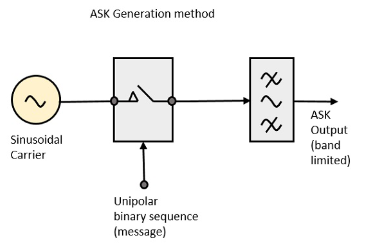1. Data source: The data source is the device or system that generates the input digital signal to be transmitted. The input digital signal can be a binary signal with two levels (0 and 1), or a multi-level signal with more than two levels.

2. Data encoder: The data encoder is a device or circuit that encodes the input digital signal into a suitable form for ASK transmission. The data encoder converts the input digital signal into a series of pulses, which represent the levels or states of the input digital signal.

3. ASK modulator: The ASK modulator is a device or circuit that modulates the encoded pulses onto a suitable carrier frequency, using a technique called amplitude shift keying (ASK). In ASK, the amplitude or level of the carrier frequency is varied according to the levels or states of the encoded pulses. For example, if the encoded pulses represent a binary signal with two levels (0 and 1), the carrier frequency would be low for a value of 0, and high for a value of 1. If the encoded pulses represent a multi-level signal with more than two levels, the carrier frequency would be set to a corresponding number of discrete levels, such as four, eight, or sixteen levels.

4. Amplifier: The amplifier is a device or circuit that amplifies the modulated carrier frequency to a suitable level for transmission. The amplifier may use a linear or nonlinear amplification technique, depending on the requirements of the ASK transmitter.

5. Antenna: The antenna is a device or structure that radiates the amplified modulated carrier frequency into the air or space, as an electromagnetic wave. The antenna may be a directional or omnidirectional antenna, depending on the coverage or range of the ASK transmitter.

This is a general description of the block diagram of an ASK transmitter. There may be additional blocks or components in the ASK transmitter, depending on the specific requirements or characteristics of the ASK transmitter.

# Recall the Bandwidth and Energy in ASK Transmission

In an amplitude shift keying (ASK) transmission, the bandwidth is the range of frequencies occupied by the transmitted signal, and the energy is the amount of power or energy transmitted by the signal.

The bandwidth of an ASK transmission is determined by the rate at which the input digital signal is encoded and modulated onto the carrier frequency. For example, if the input digital signal has a data rate of 1 kilobit per second (kbps), and the ASK modulator uses a binary signal with two levels (0 and 1), the bandwidth of the ASK transmission would be equal to the data rate of the input digital signal, or 1 kbps. If the ASK modulator uses a multi-level signal with more than two levels, the bandwidth of the ASK transmission would be greater than the data rate of the input digital signal, as more levels or states need to be transmitted.

The energy of an ASK transmission is determined by the power or energy of the carrier frequency, and the efficiency of the ASK modulator. The power or energy of the carrier frequency can be adjusted by the amplifier, and the efficiency of the ASK modulator can be improved by using a linear or nonlinear modulation technique. For example, if the carrier frequency has a power of 1 watt (W), and the ASK modulator has an efficiency of 50%, the energy of the ASK transmission would be equal to 0.5 W. If the carrier frequency has a power of 10 W, and the ASK modulator has an efficiency of 75%, the energy of the ASK transmission would be equal to 7.5 W.

These are just a few examples of the bandwidth and energy in an ASK transmission. The actual bandwidth and energy of an ASK transmission may vary, depending on the specific characteristics or requirements of the ASK transmission.

# Draw Constellation diagram of ASK

A constellation diagram is a graphical representation of a digital communication system, which shows the constellation or set of possible signal points or states of the system. In an amplitude shift keying (ASK) transmission, the constellation diagram shows the constellation or set of possible signal points or states of the ASK modulated signal.

The constellation diagram of an ASK transmission is shown below: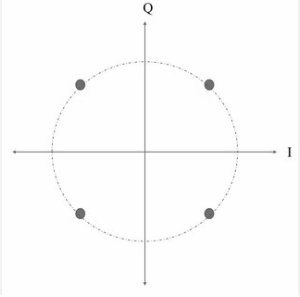In the ASK constellation diagram, the x-axis represents the in-phase component or real part of the ASK modulated signal, and the y-axis represents the quadrature component or imaginary part of the ASK modulated signal. The signal points or states of the ASK modulated signal are shown as dots or symbols on the constellation diagram, and are labeled with the corresponding levels or states of the input digital signal.

For example, if the ASK modulator uses a binary signal with two levels (0 and 1), the constellation diagram would show two signal points or states, labeled as 0 and 1. If the ASK modulator uses a multi-level signal with more than two levels, the constellation diagram would show more signal points or states, labeled with the corresponding number of levels or states.

This is a general description of the constellation diagram of an ASK transmission. The actual constellation diagram of an ASK transmission may vary, depending on the specific characteristics or requirements of the ASK transmission.

The block diagram of an amplitude shift keying (ASK) receiver is shown below: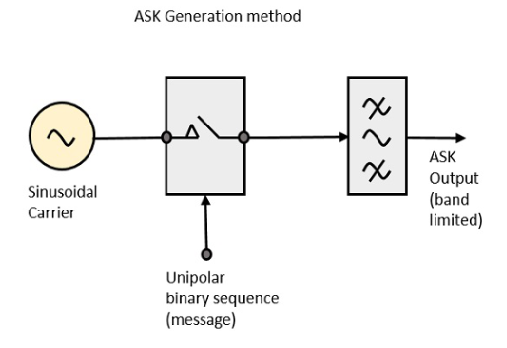1. Antenna: The antenna is a device or structure that receives the incoming ASK modulated signal as an electromagnetic wave, and converts it into an electrical signal. The antenna may be a directional or omnidirectional antenna, depending on the coverage or range of the ASK receiver.

2. Amplifier: The amplifier is a device or circuit that amplifies the incoming electrical signal to a suitable level for processing. The amplifier may use a linear or nonlinear amplification technique, depending on the requirements of the ASK receiver.

3. ASK demodulator: The ASK demodulator is a device or circuit that demodulates the incoming electrical signal, using a technique called amplitude shift keying (ASK). In ASK, the demodulator extracts the encoded pulses from the incoming electrical signal, by comparing the amplitude or level of the signal with a reference level or threshold. For example, if the encoded pulses represent a binary signal with two levels (0 and 1), the demodulator would compare the amplitude of the signal with a reference level or threshold, and output a value of 0 if the signal is below the reference level, and a value of 1 if the signal is above the reference level. If the encoded pulses represent a multi-level signal with more than two levels, the demodulator would compare the amplitude of the signal with a corresponding number of reference levels or thresholds, and output a corresponding value based on the comparison.

4. Data decoder: The data decoder is a device or circuit that decodes the output of the ASK demodulator into the original form of the input digital signal. The data decoder converts the output of the ASK demodulator into the original digital signal, by interpreting the levels or states of the encoded pulses according to the encoding scheme used by the ASK modulator.

5. Data sink: The data sink is the device or system that consumes or uses the output digital signal from the ASK receiver. The data sink may be a display, a storage device, a processing unit, or any other device or system that can handle the output digital signal.

# Describe the block diagram of FSK Transmitter

The block diagram of a frequency shift keying (FSK) transmitter is shown below: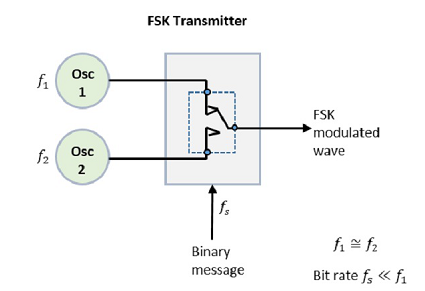1. Data source: The data source is the device or system that provides the input digital signal to the FSK transmitter. The data source may be a microphone, a keyboard, a sensor, or any other device or system that generates or captures the input digital signal.

2. Data encoder: The data encoder is a device or circuit that encodes the input digital signal into a form suitable for transmission over the communication channel. The data encoder converts the input digital signal into a series of pulses or symbols, using a technique called frequency shift keying (FSK). In FSK, the data encoder assigns different frequencies or tones to different levels or states of the input digital signal, according to the encoding scheme used by the FSK transmitter. For example, if the input digital signal represents a binary signal with two levels (0 and 1), the data encoder may assign a frequency of 1 kilohertz (kHz) to the level 0, and a frequency of 2 kHz to the level 1. If the input digital signal represents a multi-level signal with more than two levels, the data encoder may assign a corresponding number of frequencies or tones to the different levels or states.

3. FSK modulator: The FSK modulator is a device or circuit that modulates the output of the data encoder onto a carrier frequency, using a technique called frequency shift keying (FSK). In FSK, the FSK modulator shifts the frequency of the carrier frequency according to the frequencies or tones assigned by the data encoder to the different levels or states of the input digital signal. For example, if the data encoder assigns a frequency of 1 kHz to the level 0, and a frequency of 2 kHz to the level 1, the FSK modulator would shift the frequency of the carrier frequency from 1 kHz to 2 kHz, or from 2 kHz to 1 kHz, depending on the level or state of the input digital signal. If the data encoder assigns a corresponding number of frequencies or tones to the different levels or states, the FSK modulator would shift the frequency of the carrier frequency among the corresponding frequencies or tones.

4. Amplifier: The amplifier is a device or circuit that amplifies the output of the FSK modulator to a suitable level for transmission. The amplifier may use a linear or nonlinear amplification technique, depending on the requirements of the FSK transmitter.

5. Antenna: The antenna is a device or structure that radiates the output of the amplifier as an electromagnetic wave, for transmission over the communication channel. The antenna may be a directional or omnidirectional antenna, depending on the coverage or range of the FSK transmitter.

This is a general description of the block diagram of an FSK transmitter. There may be additional blocks or components in the FSK transmitter, depending on the specific requirements or characteristics of the FSK transmitter.

# Recall the Bandwidth and Energy in FSK Transmission

In a frequency shift keying (FSK) transmission, the bandwidth is the range of frequencies occupied by the FSK modulated signal. The bandwidth of an FSK transmission is determined by the frequencies or tones assigned by the data encoder to the different levels or states of the input digital signal, and the deviation or shift of the carrier frequency between the different frequencies or tones.

For example, if the data encoder assigns a frequency of 1 kilohertz (kHz) to the level 0, and a frequency of 2 kHz to the level 1, and the deviation or shift of the carrier frequency between the frequencies 1 kHz and 2 kHz is 500 hertz (Hz), the bandwidth of the FSK transmission would be 2 kHz – 1 kHz + 2 * 500 Hz = 3 kHz. If the data encoder assigns a corresponding number of frequencies or tones to the different levels or states, and the deviation or shift of the carrier frequency between the different frequencies or tones is the same, the bandwidth of the FSK transmission would be equal to the sum of the frequencies or tones assigned to the different levels or states, plus twice the deviation or shift of the carrier frequency.

The energy of an FSK transmission is the total power or energy of the FSK modulated signal, as transmitted over the communication channel. The energy of an FSK transmission is determined by the power or energy of the carrier frequency, and the deviation or shift of the carrier frequency between the different frequencies or tones.

For example, if the power or energy of the carrier frequency is 1 watt (W), and the deviation or shift of the carrier frequency between the frequencies 1 kHz and 2 kHz is 500 Hz, the energy of the FSK transmission would be equal to the power or energy of the carrier frequency, multiplied by the deviation or shift of the carrier frequency. In this case, the energy of the FSK transmission would be 1 W * 500 Hz = 0.5 W. If the deviation or shift of the carrier frequency between the different frequencies or tones is the same, the energy of the FSK transmission would be equal to the power or energy of the carrier frequency, multiplied by the deviation or shift of the carrier frequency.

This is a general description of the bandwidth and energy in an FSK transmission. The actual bandwidth and energy of an FSK transmission may vary, depending on the specific characteristics or requirements of the FSK transmission.

# Draw Constellation diagram of FSK

A constellation diagram is a graphical representation of the symbols or points in a digital modulation scheme, in the form of a two-dimensional plot, with the in-phase component (I) of the modulated signal on the x-axis, and the quadrature component (Q) of the modulated signal on the y-axis. The constellation diagram is used to visualize and analyze the characteristics of the digital modulation scheme, such as the number of symbols or points, the symmetry or distribution of the symbols or points, the distance or separation between the symbols or points, and the error rate or performance of the digital modulation scheme.

The constellation diagram of a frequency shift keying (FSK) transmission is shown below: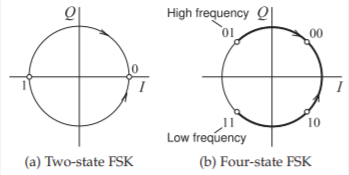In the constellation diagram of an FSK transmission, the symbols or points correspond to the frequencies or tones assigned by the data encoder to the different levels or states of the input digital signal. For example, if the data encoder assigns a frequency of 1 kilohertz (kHz) to the level 0, and a frequency of 2 kHz to the level 1, the symbols or points in the constellation diagram of the FSK transmission would represent the frequencies 1 kHz and 2 kHz, as shown in the diagram above. If the data encoder assigns a corresponding number of frequencies or tones to the different levels or states, the symbols or points in the constellation diagram of the FSK transmission would represent the corresponding frequencies or tones.

In the constellation diagram of an FSK transmission, the distance or separation between the symbols or points is a measure of the error rate or performance of the FSK transmission. The larger the distance or separation between the symbols or points, the lower the error rate or performance of the FSK transmission. The smaller the distance or separation between the symbols or points, the higher the error rate or performance of the FSK transmission.

This is a general description of the constellation diagram of an FSK transmission. The actual constellation diagram of an FSK transmission may vary, depending on the specific characteristics or requirements of the FSK transmission.

# Describe the block diagram of FSK Receiver

A block diagram of a frequency shift keying (FSK) receiver is shown below: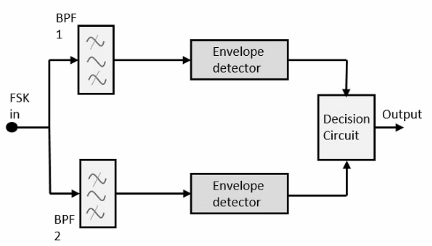The FSK receiver consists of the following main blocks or stages:

1. Antenna: The antenna is a device that receives the FSK modulated signal from the communication channel, and converts the electromagnetic waves of the FSK modulated signal into electrical signals.

2. RF Amplifier: The RF amplifier is a circuit that amplifies the electrical signals from the antenna, and prepares the signals for the next stage of the FSK receiver.

3. Bandpass Filter: The bandpass filter is a circuit that filters out or removes the unwanted or out-of-band frequencies from the amplified signals, and passes or allows the wanted or in-band frequencies of the FSK modulated signal.

4. Frequency Discriminator: The frequency discriminator is a circuit that separates or demodulates the frequency or tone of the FSK modulated signal, based on the deviation or shift of the carrier frequency between the different frequencies or tones.

5. Data Decoder: The data decoder is a circuit that decodes or recovers the data or information from the frequency or tone of the FSK modulated signal, based on the frequencies or tones assigned by the data encoder to the different levels or states of the input digital signal.

6. Output: The output of the FSK receiver is the data or information decoded or recovered from the FSK modulated signal.

This is a general description of the block diagram of an FSK receiver. The actual block diagram of an FSK receiver may vary, depending on the specific characteristics or requirements of the FSK receiver.

# Describe the block diagram of PSK Transmitter

A block diagram of a phase shift keying (PSK) transmitter is shown below: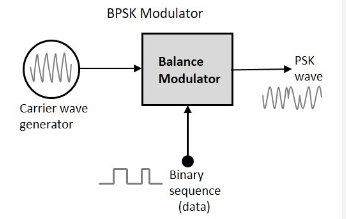The PSK transmitter consists of the following main blocks or stages:

1. Data Encoder: The data encoder is a circuit that encodes or converts the data or information into a digital signal, based on the levels or states of the data or information.

2. Phase Modulator: The phase modulator is a circuit that modulates or shifts the phase of a carrier frequency, based on the digital signal from the data encoder.

3. RF Amplifier: The RF amplifier is a circuit that amplifies the modulated signal from the phase modulator, and prepares the signal for transmission.

4. Antenna: The antenna is a device that transmits the modulated signal from the RF amplifier, and converts the electrical signals of the modulated signal into electromagnetic waves.

This is a general description of the block diagram of a PSK transmitter. The actual block diagram of a PSK transmitter may vary, depending on the specific characteristics or requirements of the PSK transmitter.

# Recall the Bandwidth and Energy in PSK Transmission

In a phase shift keying (PSK) transmission, the bandwidth is the range or span of frequencies occupied or used by the modulated signal. The bandwidth of a PSK transmission depends on the data rate or the number of bits per second transmitted by the PSK transmitter, and the modulation index or the deviation or shift of the carrier phase between the different phases or states of the PSK modulated signal.

In a PSK transmission, the energy is the power or intensity of the modulated signal, measured in watts (W) or decibels (dB). The energy of a PSK transmission depends on the data rate or the number of bits per second transmitted by the PSK transmitter, and the modulation index or the deviation or shift of the carrier phase between the different phases or states of the PSK modulated signal.

The bandwidth and energy of a PSK transmission are related, in that a larger bandwidth or a higher data rate results in a higher energy or a higher power of the modulated signal, and a smaller bandwidth or a lower data rate results in a lower energy or a lower power of the modulated signal.

This is a general description of the bandwidth and energy in a PSK transmission. The actual bandwidth and energy of a PSK transmission may vary, depending on the specific characteristics or requirements of the PSK transmission.

# Draw Constellation diagram of PSK

A constellation diagram of a phase shift keying (PSK) modulation is a graphical representation of the different phases or states of the PSK modulated signal, based on the deviation or shift of the carrier phase between the different phases or states.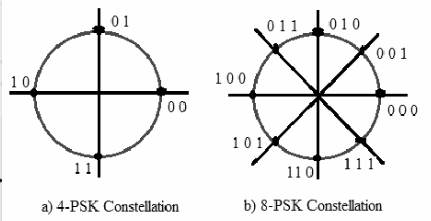In a constellation diagram of a PSK modulation, the x-axis represents the in-phase (I) component or the real part of the PSK modulated signal, and the y-axis represents the quadrature (Q) component or the imaginary part of the PSK modulated signal. The constellation points or symbols in the diagram represent the different phases or states of the PSK modulated signal, based on the deviation or shift of the carrier phase between the different phases or states.

For example, in a binary phase shift keying (BPSK) modulation, the PSK modulated signal has two phases or states, corresponding to two constellation points or symbols at 0 degrees and 180 degrees of the carrier phase. In a quadrature phase shift keying (QPSK) modulation, the PSK modulated signal has four phases or states, corresponding to four constellation points or symbols at 0 degrees, 90 degrees, 180 degrees, and 270 degrees of the carrier phase.

This is a general description of the constellation diagram of a PSK modulation. The actual constellation diagram of a PSK modulation may vary, depending on the specific characteristics or requirements of the PSK modulation.

# Describe the block diagram of PSK Receiver

A block diagram of a phase shift keying (PSK) receiver is shown below: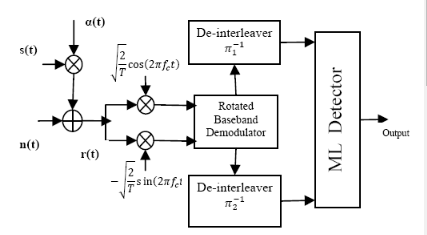The PSK receiver consists of the following main blocks or stages:

1. Antenna: The antenna is a device that receives the PSK modulated signal from the communication channel, and converts the electromagnetic waves of the PSK modulated signal into electrical signals.

2. RF Amplifier: The RF amplifier is a circuit that amplifies the electrical signals from the antenna, and prepares the signals for the next stage of the PSK receiver.

3. Bandpass Filter: The bandpass filter is a circuit that filters out or removes the unwanted or out-of-band frequencies from the amplified signals, and passes or allows the wanted or in-band frequencies of the PSK modulated signal.

4. Phase Discriminator: The phase discriminator is a circuit that separates or demodulates the phase or state of the PSK modulated signal, based on the deviation or shift of the carrier phase between the different phases or states.

5. Data Decoder: The data decoder is a circuit that decodes or recovers the data or information from the phase or state of the PSK modulated signal, based on the phases or states assigned by the data encoder to the different levels or states of the input digital signal.

6. Output: The output of the PSK receiver is the data or information decoded or recovered from the PSK modulated signal.

This is a general description of the block diagram of a PSK receiver. The actual block diagram of a PSK receiver may vary, depending on the specific characteristics or requirements of the PSK receiver.

# Describe the block diagram of QPSK Transmitter

A block diagram of a quadrature phase shift keying (QPSK) transmitter is shown below: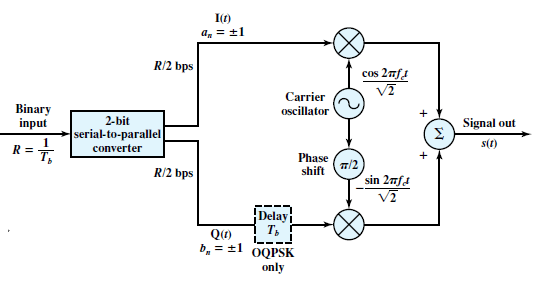The QPSK transmitter consists of the following main blocks or stages:

1. Data Encoder: The data encoder is a circuit that encodes or converts the data or information into a digital signal, based on the levels or states of the data or information.

2. Quadrature Modulator: The quadrature modulator is a circuit that modulates or shifts the phase of a carrier frequency, based on the digital signal from the data encoder. The quadrature modulator consists of two phase modulators, one for the in-phase (I) component or the real part of the QPSK modulated signal, and one for the quadrature (Q) component or the imaginary part of the QPSK modulated signal.

3. RF Amplifier: The RF amplifier is a circuit that amplifies the modulated signal from the quadrature modulator, and prepares the signal for transmission.

4. Antenna: The antenna is a device that transmits the modulated signal from the RF amplifier, and converts the electrical signals of the modulated signal into electromagnetic waves.

This is a general description of the block diagram of a QPSK transmitter. The actual block diagram of a QPSK transmitter may vary, depending on the specific characteristics or requirements of the QPSK transmitter.

# Recall the Bandwidth and Energy in QPSK Transmission

In QPSK (Quadrature Phase Shift Keying) transmission, the bandwidth required for the transmission of a given data rate is determined by the symbol rate, which is the number of symbols transmitted per second. Each symbol in QPSK represents two bits of information, so the data rate is twice the symbol rate.

For example, if the symbol rate is 1 mega symbol per second (Msps), then the data rate is 2 megabits per second (Mbps). The bandwidth required for QPSK transmission at this symbol rate would be the symbol rate, or 1 MHz.

The energy required for QPSK transmission depends on the power of the transmitted signal and the distance over which the signal is being transmitted. In general, the energy required for transmission increases with the distance over which the signal is being transmitted, as the signal must be transmitted with sufficient power to overcome losses due to free space propagation, atmospheric absorption, and other factors. The energy required also increases with the power of the transmitted signal, as more energy is required to transmit a stronger signal.

# Draw Constellation diagram of QPSK

In QPSK (Quadrature Phase Shift Keying), each symbol is represented by a point on a constellation diagram, which is a graphical representation of the possible symbol values. The constellation diagram for QPSK is shown below: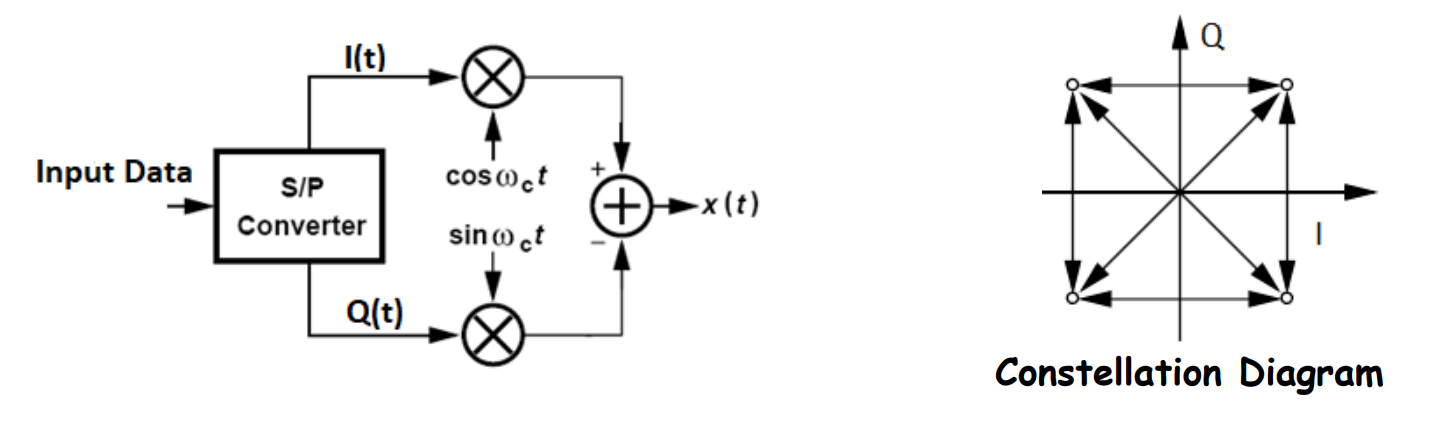Each point on the constellation diagram represents a unique combination of two bits of information. The four points on the diagram represent the four possible symbol values: 00, 01, 10, and 11.

In QPSK, the phase of the transmitted signal is changed to represent the different symbol values. For example, a symbol value of 00 might be represented by a phase of 0 degrees, a symbol value of 01 might be represented by a phase of 90 degrees, a symbol value of 10 might be represented by a phase of 180 degrees, and a symbol value of 11 might be represented by a phase of 270 degrees.

The constellation diagram for QPSK can be used to visualize the transmitted signal and to diagnose any errors that may occur in the transmission process. It is often used in the design and analysis of communication systems that use QPSK.

# Describe the block diagram of QPSK Receiver

A Quadrature Phase Shift Keying (QPSK) receiver is a digital communication system that demodulates a QPSK signal to extract the transmitted data. The block diagram of a QPSK receiver typically consists of the following blocks: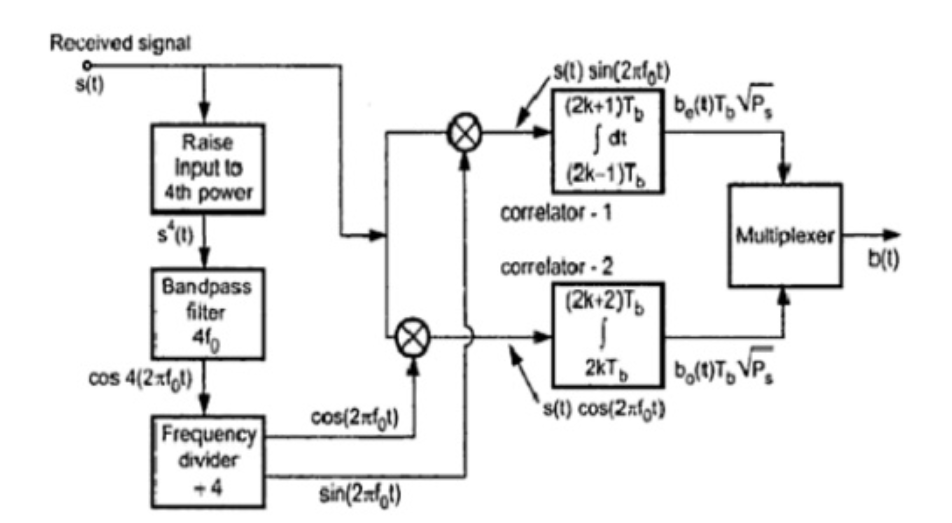1. Low-Noise Amplifier (LNA): The received QPSK signal is weak and needs to be amplified before processing. The Low-Noise Amplifier (LNA) amplifies the received signal while adding as little noise as possible.
2. Mixer: The Mixer mixes the amplified QPSK signal with a local oscillator signal to produce an intermediate frequency (IF) signal. The IF signal is centered at a frequency that is lower than the carrier frequency of the original QPSK signal.
3. Bandpass Filter: The Bandpass Filter filters out unwanted signals that may have been picked up during transmission or amplification. The filter only allows the desired IF signal to pass through to the next stage.
4. Automatic Gain Control (AGC): The Automatic Gain Control (AGC) adjusts the gain of the IF signal to a constant level, which is necessary for the subsequent demodulation process.
5. Phase-Locked Loop (PLL): The Phase-Locked Loop (PLL) generates a stable reference signal that is in phase with the carrier of the transmitted QPSK signal. This reference signal is used for demodulation.
6. Demodulator: The Demodulator separates the in-phase and quadrature components of the IF signal and compares them with the reference signal generated by the PLL. This process recovers the original data that was transmitted using QPSK modulation.
7. Decoder: The Decoder converts the demodulated digital signal into the original message signal that was transmitted.
8. Error Correction: The Error Correction block checks the decoded message signal for errors and corrects them if possible. This block is optional but is often included to improve the reliability of the system.
9. Output: The Output block provides the final output of the QPSK receiver, which is the recovered message signal with or without error correction. This signal can be further processed for display or storage.

# Recall the block diagram of DPSK Transmitter

A Differential Phase Shift Keying (DPSK) transmitter is a digital communication system that modulates data onto a carrier signal using changes in the phase of the signal. The block diagram of a DPSK transmitter typically consists of the following blocks:

DPSK Modulator diagram: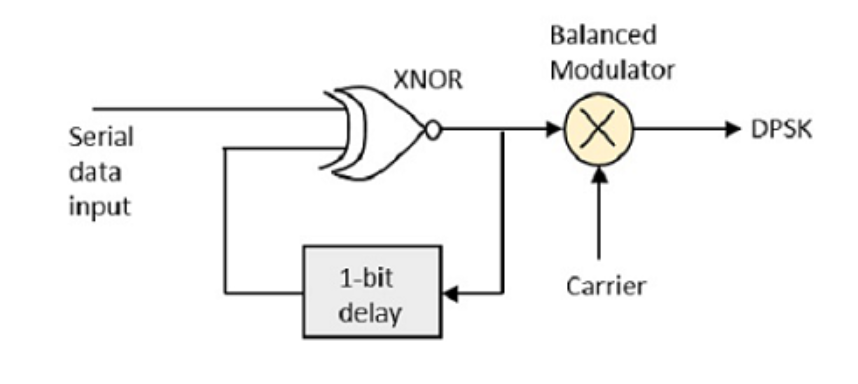1. Input: The Input block is where the data to be transmitted is fed into the system.
2. Digital Encoder: The Digital Encoder converts the input data into a binary sequence that can be modulated onto the carrier signal.
3. Differential Encoder: The Differential Encoder generates a new binary sequence by taking the XOR of the current bit with the previous bit. This generates a sequence of symbols that can be used to modulate the carrier signal.
4. Modulator: The Modulator modulates the carrier signal by changing its phase according to the symbols generated by the Differential Encoder. In DPSK, the phase shift is relative to the previous symbol, so the modulator changes the phase by either 0 or 180 degrees, depending on the value of the current symbol.
5. Amplifier: The Amplifier boosts the power of the modulated signal to a level appropriate for transmission.
6. Antenna: The Antenna transmits the modulated signal over the airwaves to the receiver.

Model waveform of DPSK: